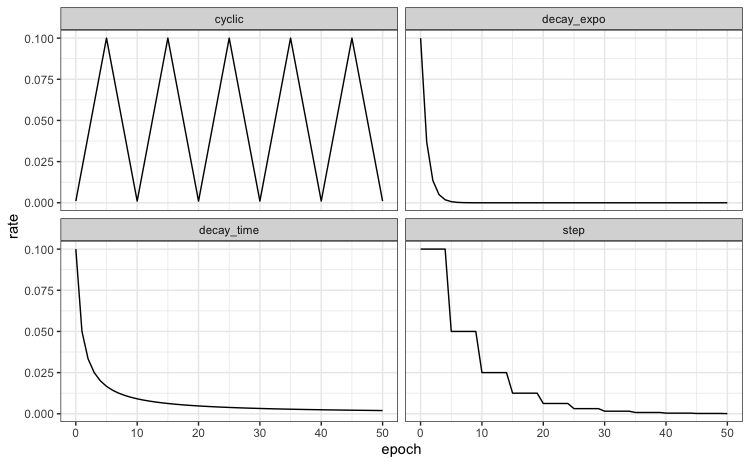# brulee 0.2.0

Max Kuhn

We’re thrilled to announce the release of brulee 0.2.0. brulee contains several basic modeling functions that use the torch package infrastructure, such as: neural networks, linear regression, logistic regression, and multinomial regression.

You can install it from CRAN with:

install.packages("brulee")


This blog post will describe the changes to the package. You can see a full list of changes in the release notes.

There were two main additions to brulee.

First, since brulee is focused on fitting models to tabular data, we have moved away from optimizing via stochastic gradient descent (SGD) as the default. For brulee_mlp(), we switched the default optimizer from SGD to more traditional quasi-newton methods, specifically to Broyden–Fletcher–Goldfarb–Shanno algorithm (BFGS) method. You can still use SGD via the optimizer option.

Second, we’ve added learning rate schedulers to brulee_mlp(). The learning rate is one of the most important parameters to tune. There is an existing option to have a constant learning rate (via the learn_rate argument). However, there is some intuition that the rate should probably decrease once the optimizer is closer to the best solution (to avoid overshooting the target). A scheduler is a function that adjusts the rate over time. Apart from a constant learning rate (the default), the options are cyclic, exponential decay, time-based decay, and step functions:The corresponding set of functions share the prefix schedule_*().

To use these with brulee_mlp(), there is a rate_schedule argument with possible values: "none" (the default), "decay_time", "decay_expo", "cyclic" and "step". Each function has arguments and these can be passed directly to brulee_mlp(). The rate_schedule argument can also be tuned as any other engine-specific parameter.

## An example

Let’s look at an example using the Ames housing data. We’ll use tidymodels to split the data and also preprocess the data a bit.

library(tidymodels)
library(brulee)

# ------------------------------------------------------------------------------

tidymodels_prefer()
theme_set(theme_bw())

# ------------------------------------------------------------------------------

data(ames, package = "modeldata")
ames$Sale_Price <- log10(ames$Sale_Price)

# ------------------------------------------------------------------------------

set.seed(5685)
split <- initial_split(ames)
ames_train <- training(split)
ames_test  <- testing(split)

# ------------------------------------------------------------------------------
# Let's make a recipe to preprocess the data

ames_rec <-
recipe(Sale_Price ~ Bldg_Type + Neighborhood + Year_Built + Gr_Liv_Area +
Full_Bath + Year_Sold + Lot_Area + Central_Air + Longitude + Latitude,
data = ames_train) %>%
# Transform some highly skewed predictors
step_BoxCox(Lot_Area, Gr_Liv_Area) %>%
# Lump some rarely occurring categories into "other"
step_other(Neighborhood, threshold = 0.05)  %>%
# Encode categorical predictors as binary.
step_dummy(all_nominal_predictors(), one_hot = TRUE) %>%
step_interact(~ starts_with("Central_Air"):Year_Built) %>%
step_zv(all_predictors()) %>%
step_normalize(all_numeric_predictors())


Now we can fit the model by passing the data, the recipe, and other options to brulee_mlp(). We’ll use a cyclic scheduler with a half-cycle size of 5 epochs:

set.seed(827)
fit <- brulee_mlp(ames_rec, data = ames_train, hidden_units = 20, epochs = 151,
penalty = 0.05, rate_schedule = "cyclic", step_size = 5)

# Show the validation loss and alter the x-axis tick marks to correspond to cycles.
cycles <- seq(1, 151, by = 10)
autoplot(fit) + scale_x_continuous(breaks = cycles, minor_breaks = NULL)## Acknowledgements

We’d like to thank @EmilHvitfeldt, @sametsoekel, and @dfalbel for their help since the previous release.

Contents